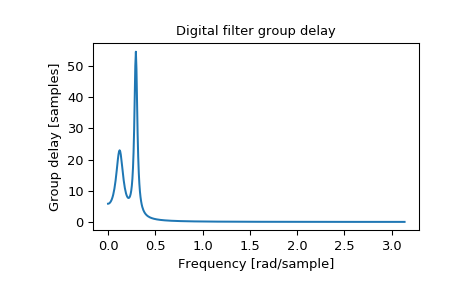# scipy.signal.group_delay¶

scipy.signal.group_delay(system, w=512, whole=False, fs=6.283185307179586)[source]

Compute the group delay of a digital filter.

The group delay measures by how many samples amplitude envelopes of various spectral components of a signal are delayed by a filter. It is formally defined as the derivative of continuous (unwrapped) phase:

          d        jw
D(w) = - -- arg H(e)
dw

Parameters
systemtuple of array_like (b, a)

Numerator and denominator coefficients of a filter transfer function.

w{None, int, array_like}, optional

If a single integer, then compute at that many frequencies (default is N=512).

If an array_like, compute the delay at the frequencies given. These are in the same units as fs.

wholebool, optional

Normally, frequencies are computed from 0 to the Nyquist frequency, fs/2 (upper-half of unit-circle). If whole is True, compute frequencies from 0 to fs. Ignored if w is array_like.

fsfloat, optional

The sampling frequency of the digital system. Defaults to 2*pi radians/sample (so w is from 0 to pi).

New in version 1.2.0.

Returns
wndarray

The frequencies at which group delay was computed, in the same units as fs. By default, w is normalized to the range [0, pi) (radians/sample).

gdndarray

The group delay.

freqz

Frequency response of a digital filter

Notes

The similar function in MATLAB is called grpdelay.

If the transfer function $$H(z)$$ has zeros or poles on the unit circle, the group delay at corresponding frequencies is undefined. When such a case arises the warning is raised and the group delay is set to 0 at those frequencies.

For the details of numerical computation of the group delay refer to .

New in version 0.16.0.

References

1(1,2)

Richard G. Lyons, “Understanding Digital Signal Processing, 3rd edition”, p. 830.

Examples

>>> from scipy import signal
>>> b, a = signal.iirdesign(0.1, 0.3, 5, 50, ftype='cheby1')
>>> w, gd = signal.group_delay((b, a))

>>> import matplotlib.pyplot as plt
>>> plt.title('Digital filter group delay')
>>> plt.plot(w, gd)
>>> plt.ylabel('Group delay [samples]')